axis_geo behaves similarly to axis in that it adds an axis to the specified side of a base R plot. The main difference is that it also adds a geological timescale between the plot and the axis. The default scale includes international epochs from the the Geological Timescale 2020 (GTS2020). However, international stages, periods, eras, and eons are also available. Interval data hosted by Macrostrat are also available (see time_bins). A custom interval dataset can also be used (see Details below). The appearance of the axis is highly customisable (see Usage below), with the intent that plots will be publication-ready.

## Usage

axis_geo(
side = 1,
intervals = "epoch",
height = 0.05,
fill = NULL,
lab = TRUE,
lab_col = NULL,
lab_size = 1,
rot = 0,
abbr = TRUE,
center_end_labels = TRUE,
skip = c("Quaternary", "Holocene", "Late Pleistocene"),
bord_col = "black",
lty = par("lty"),
lwd = par("lwd"),
bkgd = "grey90",
neg = FALSE,
exact = FALSE,
round = FALSE,
tick_at = NULL,
tick_labels = TRUE,
phylo = FALSE,
root.time = NULL,
...
)

axis_geo_phylo(...)

## Arguments

side

integer. Which side to add the axis to (1: bottom, the default; 2: left; 3: top; 4: right).

intervals

The interval information to use to plot the axis: either A) a character string indicating a rank of intervals from the built-in GTS2020, B) a character string indicating a data.frame hosted by Macrostrat (see time_bins), or C) a custom data.frame of time interval boundaries (see Details). A list of strings or data.frames can be supplied to add multiple time scales to the same side of the plot (see Details).

height

numeric. The relative height (or width if side is 2 or 4) of the scale. This is relative to the height (if side is 1 or 3) or width (if side is 2 or 4) of the plot.

fill

character. The fill colour of the boxes. The default is to use the colour or color column included in intervals. If a custom dataset is provided with intervals without a colour or color column and without specifying fill, a greyscale will be used. Custom fill colours can be provided with this option (overriding the colour or color column) and will be recycled if/as necessary.

lab

logical. Should interval labels be included?

lab_col

character. The colour of the labels. The default is to use the font or lab_color column included in intervals. If a custom dataset is provided with intervals without a font or lab_color column and without specifying lab_col, all labels will be black. Custom label colours can be provided with this option (overriding the font or lab_color column) and will be recycled if/as necessary.

lab_size

numeric. The size of the labels (see cex in graphics parameters).

rot

numeric. The amount of counter-clockwise rotation to add to the labels (in degrees). Note, labels for axes added to the left or right sides are already rotated 90 degrees.

abbr

logical. Should labels be abbreviated? This only works if the data has an abbr column, otherwise the name column will be used regardless of this setting.

center_end_labels

logical. Should labels be centered within the visible range of intervals at the ends of the axis?

skip

A character vector of interval names indicating which intervals should not be labeled. If abbr is TRUE, this can also include interval abbreviations. Quaternary, Holocene, and Late Pleistocene are skipped by default. Set to NULL if this is not desired.

bord_col

character. The border colour of the interval boxes.

lty

character. Line type (see lty in graphics parameters). This value (or the last value if this is a list) will also be passed to axis.

lwd

numeric. Line width (see lwd in graphics parameters).

bkgd

character. The colour of the background of the scale when no intervals are being shown.

neg

logical. Set this to TRUE if your x-axis is using negative values. If the entire axis is already negative, this will be set to TRUE for you.

exact

logical. Set this to TRUE if you want axis tick marks and numeric tick labels placed at the interval boundaries. If TRUE, this overrides tick_at and tick_labels.

round

integer. Number of decimal places to which exact axis labels should be rounded (using round). If no value is specified, the exact values will be used. Trailing zeros are always removed. tick_at and tick_labels can be used to include labels with trailing zeros.

tick_at

A numeric vector specifying custom points at which tick marks are to be drawn on the axis. If specified, this is passed directly to axis. If phylo is TRUE, these values are converted as necessary for the phylogenetic axis limits. If this is set to NULL (the default) tick mark locations are computed automatically (see axTicks).

tick_labels

Either a) a logical value specifying whether (numerical) annotations should be made at the tick marks specified by tick_at, or b) a custom character or expression vector of labels to be placed at the tick marks. If tick_at is specified, this argument is passed directly to axis.

phylo

logical. Is the base plot a phylogeny generated by plot.phylo, plotTree, plotSimmap, etc?

root.time

numeric. If phylo is TRUE, this is the time assigned to the root node of the tree. By default, this is taken from the root.time element of the plotted tree.

...

Further arguments that are passed directly to axis.

## Value

No return value. Function is used for its side effect, which is to add an axis of the geological timescale to an already existing plot.

## Details

If a custom data.frame is provided (with intervals), it should consist of at least 3 columns of data. See GTS2020 for an example.

• The interval_name column (name is also allowed) lists the names of each time interval. These will be used as labels if no abbreviations are provided.

• The max_ma column (max_age is also allowed) lists the oldest boundary of each time interval. Values should always be positive.

• The min_ma column (min_age is also allowed) lists the youngest boundary of each time interval. Values should always be positive.

• The abbr column is optional and lists abbreviations that may be used as labels.

• The colour column (color is also allowed) is also optional and lists a colour for the background for each time interval (see the Color Specification section here).

• The font (lab_color is also allowed) column is also optional and lists a colour for the label for each time interval (see the Color Specification section here).

intervals may also be a list if multiple time scales should be added to a single side of the plot. In this case, height, fill, lab, lab_col, lab_size, rot, abbr, center_end_labels, skip, bord_col, lty, and lwd can also be lists. If these lists are not as long as intervals, the elements will be recycled. If individual values (or vectors, e.g. for skip) are used for these parameters, they will be applied to all time scales (and recycled as necessary). If multiple scales are requested they will be added sequentially outwards starting from the plot border. The axis will always be placed on the outside of the last scale.

If you would like to use intervals from the Geological Time Scale 2012 (GTS2012), you can use time_bins and supply the returned data.frame to the intervals argument.

axis_geo_phylo(...) is shorthand for axis_geo(..., phylo = TRUE).

## Authors

William Gearty & Kilian Eichenseer

Lewis A. Jones

## Examples

# track user par
# single scale on bottom
par(mar = c(6.1, 4.1, 4.1, 2.1)) # modify margin
plot(0:100, axes = FALSE, xlim = c(100, 0), ylim = c(100, 0),
xlab = NA, ylab = "Depth (m)")
box()
axis(2)
axis_geo(side = 1, intervals = "period")
# the line argument here depends on the absolute size of the plot
title(xlab = "Time (Ma)", line = 4)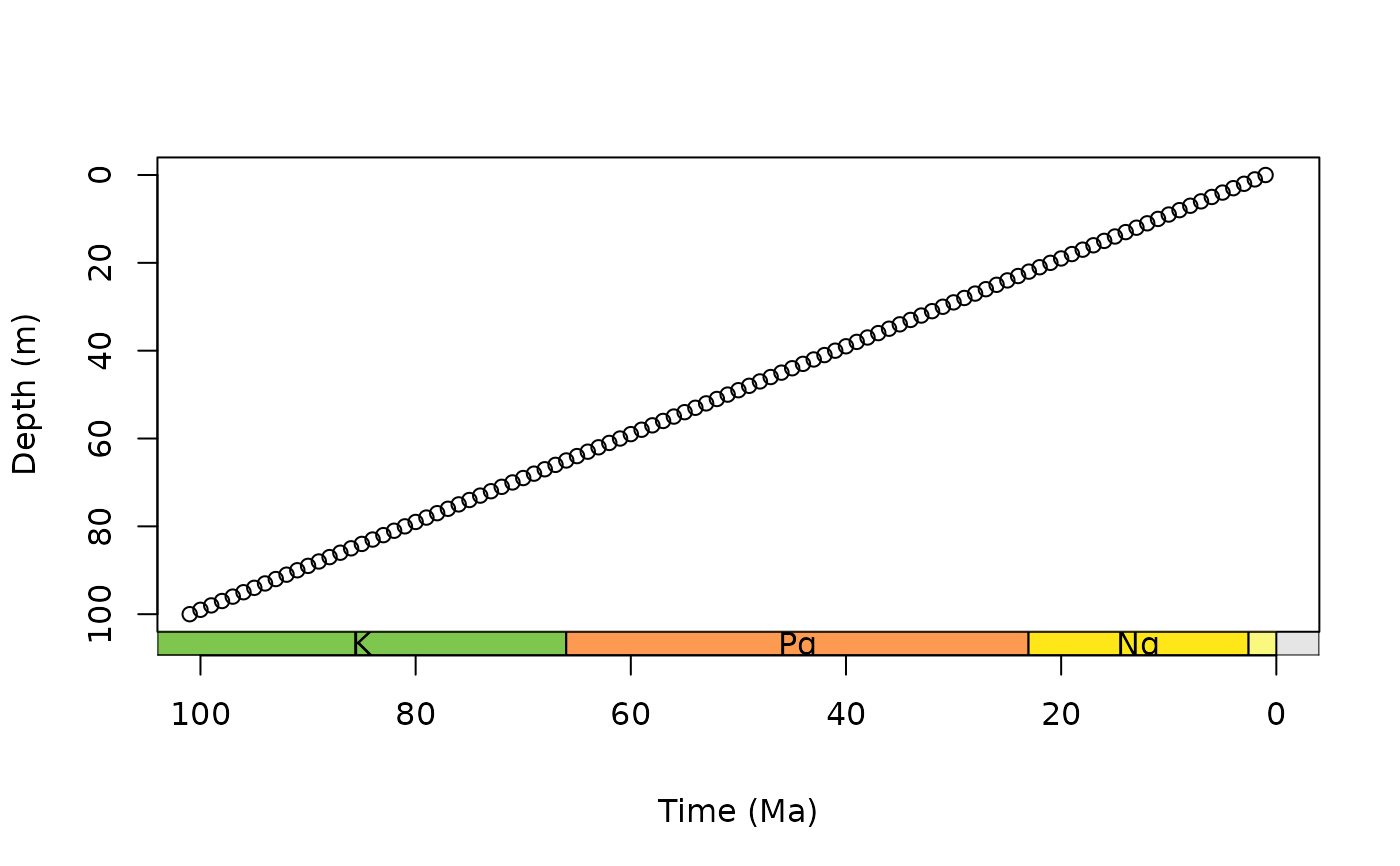# stack multiple scales, abbreviate only one set of labels
par(mar = c(7.1, 4.1, 4.1, 2.1)) # further expand bottom margin
plot(0:100, axes = FALSE, xlim = c(100, 0), ylim = c(100, 0),
xlab = NA, ylab = "Depth (m)")
box()
axis(2)
axis_geo(side = 1, intervals = list("epoch", "period"),
abbr = list(TRUE, FALSE))
# the line argument here depends on the absolute size of the plot
title(xlab = "Time (Ma)", line = 6)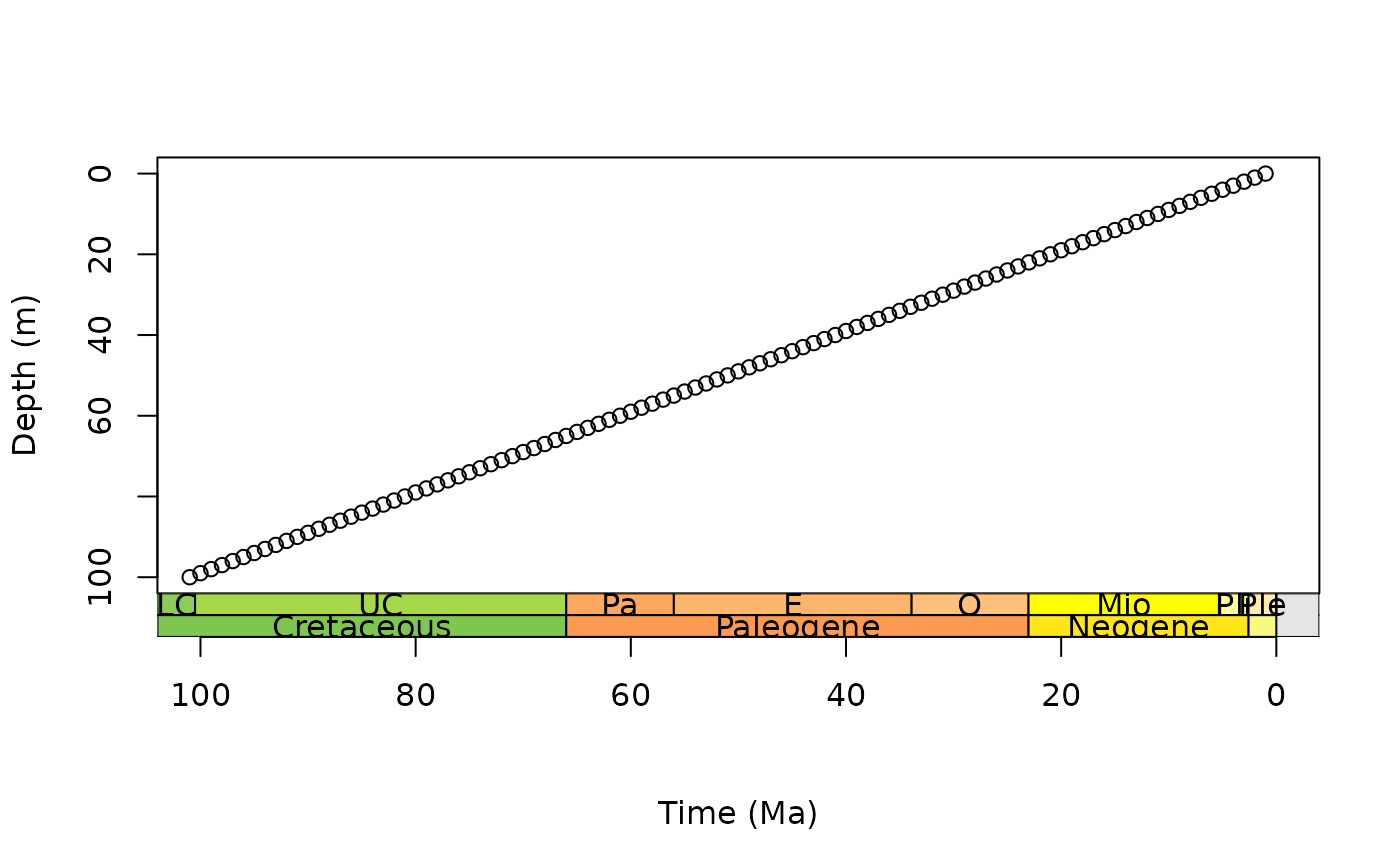# scale with MacroStrat intervals
par(mar = c(6.1, 4.1, 4.1, 2.1)) # modify margin
plot(0:30, axes = FALSE, xlim = c(30, 0), ylim = c(30, 0),
xlab = NA, ylab = "Depth (m)")
box()
axis(2)
axis_geo(side = 1, intervals = "North American land mammal ages")
# the line argument here depends on the absolute size of the plot
title(xlab = "Time (Ma)", line = 4)# scale with custom intervals
intervals <- data.frame(min_ma = c(0, 10, 25, 32),
max_ma = c(10, 25, 32, 40),
interval_name = c("A", "B", "C", "D"))
par(mar = c(6.1, 4.1, 4.1, 2.1)) # modify margin
plot(0:40, axes = FALSE, xlim = c(40, 0), ylim = c(40, 0),
xlab = NA, ylab = "Depth (m)")
box()
axis(2)
axis_geo(side = 1, intervals = intervals)
# the line argument here depends on the absolute size of the plot
title(xlab = "Time (Ma)", line = 4)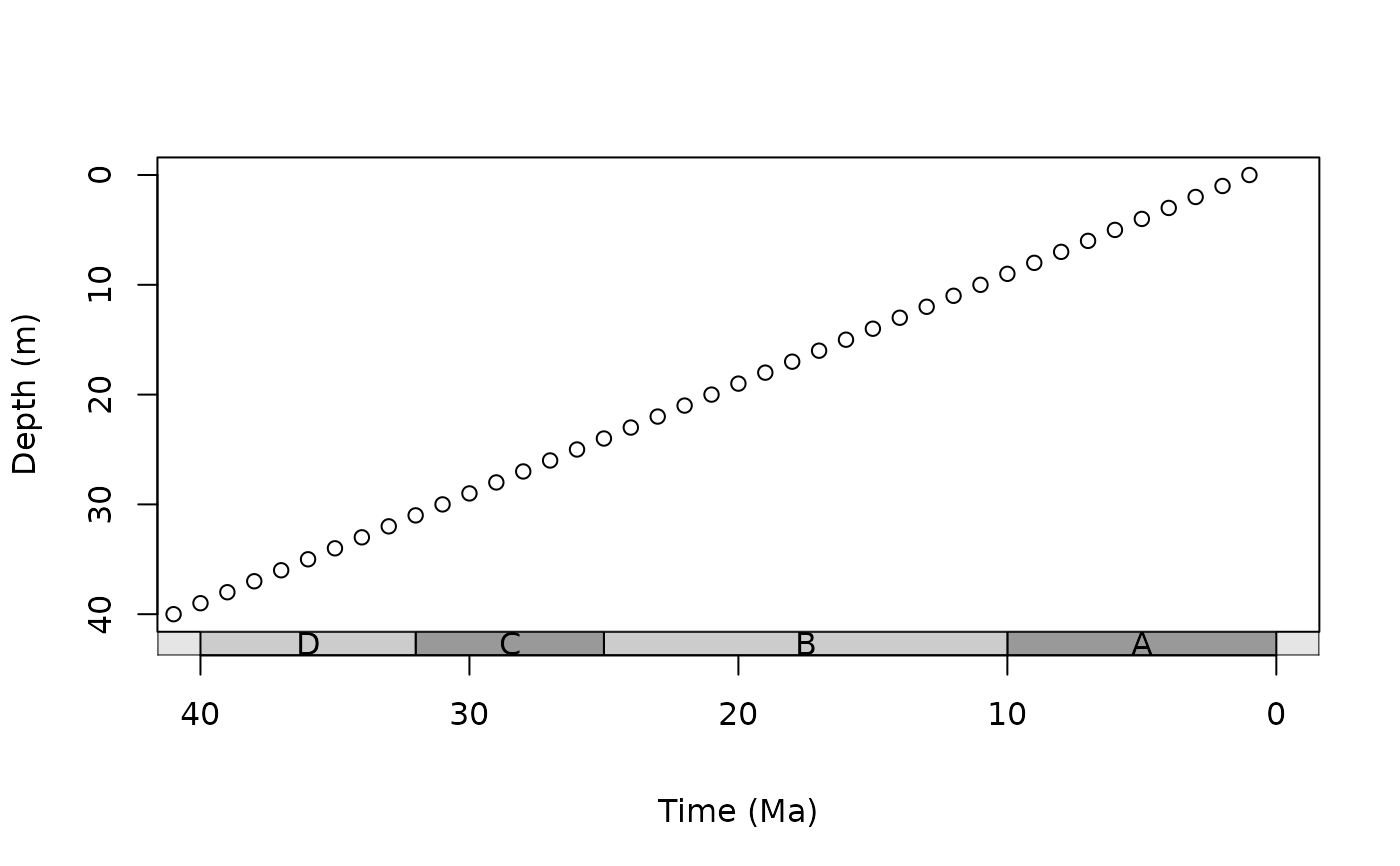# scale with phylogeny
library(phytools)
data(mammal.tree)
plot(mammal.tree)
axis_geo_phylo()
title(xlab = "Time (Ma)", line = 4)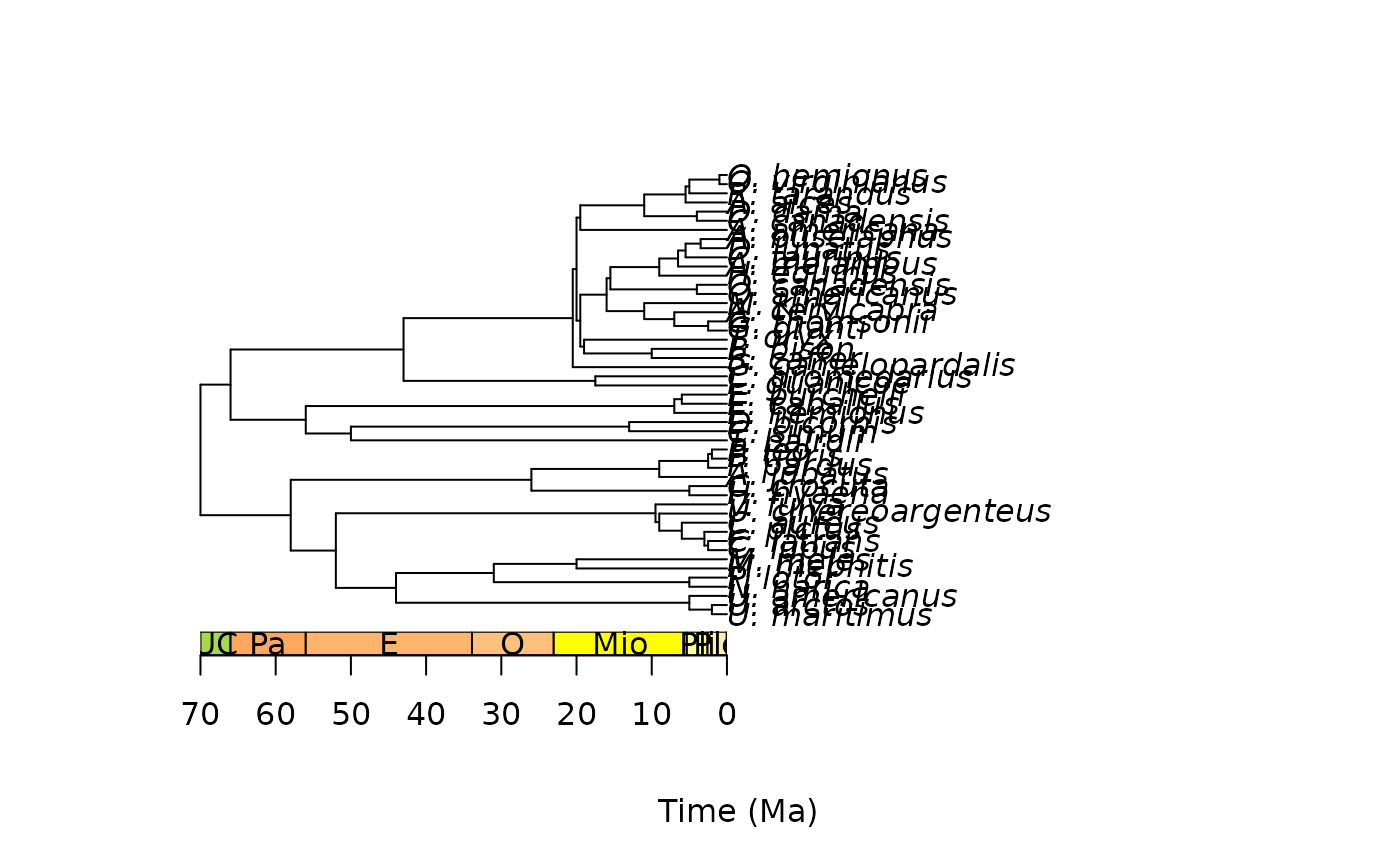# scale with fossil phylogeny
library(paleotree)
data(RaiaCopesRule)
plot(ceratopsianTreeRaia)
axis_geo_phylo()
title(xlab = "Time (Ma)", line = 4)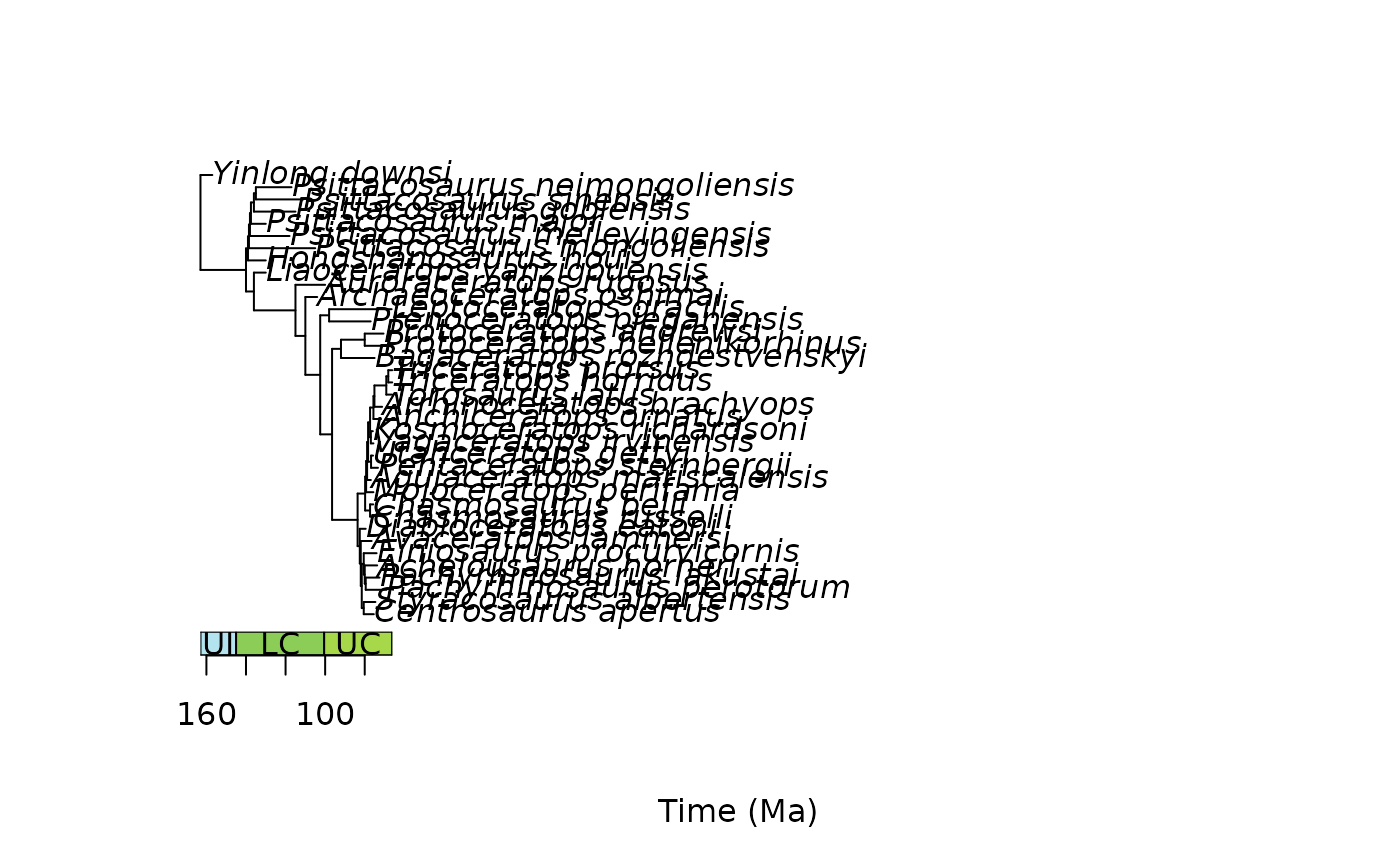# reset user par
par(oldpar)